# Time - math word problems

#### Number of problems found: 815

• Worker's performance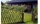15 workers paint 180 m fence in 3 days. In how many days will 9 workers paint a 360 m fence? We assume that each worker have the same, constant and unchangeable performance.
• Self-oscillation period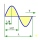The water in the vessel carried by the boy has a self-oscillation period of 0.8 s. What is the size of the boy's movement speed when the length of the boy's step is 60 cm? Give the result in m/s.
• Six studentsTwo pupils painted the class in four hours. How long will it take for six pupils?
• Clock handsCalculate the internal angles of a triangle whose vertices lie on the clock's 2, 6 and 11 hours.
• In the bakery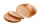In 2.25 hours, 24 pieces of bread are baked in the bakery. How many breads are baked in the bakery in 6 hours and 45 minutes?
• Train delay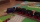Due to a breakdown, the train lost 16 minutes of standing on the track behind Brno. He "eliminated" this delay so that after the start, the 80 km long section went at a speed 10 km/h higher than originally planned. What speed was it and what was it suppos
• Growth of woodThe annual growth of wood in the forest is estimated at 2%. In how many years will make the forest volume double?
• Father and sons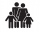Father is 27 and his sons 2 and one year. In how many years will his sons sum up to half his age?
• Age ratioJanko is 14 years old. The age ratio of Janka and Zuzka is 2: 3. What was the ratio seven years ago?
• Two dogs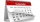Izzy's dog is 10 1/2 years old. Paige's dog is 18 months old. How many years older is Izzy's dog?
• Five harvests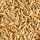In the seed company, they know that, out of 100 grains of a new variety, they get an average of 2000 grains after harvest. Approximately how many grains do they get out of 100 grains after five harvests?
• Panel house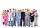The construction company has two groups of workers. Group A has more members than B. Panel house was insulated by group A in 10 days. The same block of flats was insulated together in 6 days. How many days would group B block insulate?
• Two portsFrom port A on the river, the steamer started at an average speed of 12 km/h towards port B. Two hours later, another steamer departed from A at an average speed of 20 km/h. Both ships arrived in B at the same time. What is the distance between ports A an
• AgesFather is 36 years old, his daughter is 4 years old. Write down the ratio of the age of father and daughter. In what ratio will the ages of father and daughter after 4 years?
• Magnitude of angleWhat magnitude has an obtuse angle enclosed by the hands of clocks at 12:20 hours?
• Rate 3A liquid is heated at a rate of 5 degrees Celsius per minute. If the temperature now is at 39 degrees Celsius, after how long will the temperature be 94 degrees Celsius?
• Volume of woodEvery year, at the same time, an increase in the volume of wood in the forest is measured. The increase is regularly p% compared to the previous year. If in 10 years the volume of wood has increased by 10%, what is the number p?
• FamilyThe twins Nina and Ema have five years younger brother Michal. Together have 43 years. How old is Michal?
• Mary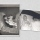Mary is 12 years old, which is 40% of the age of her mother. How many percent of her mother's age will Mary have after the next 12 years?
• Doug bikedDoug biked 5 1/4 miles in 3/4 of an hour. What is his average speed?

Do you have an interesting mathematical word problem that you can't solve it? Submit a math problem, and we can try to solve it.

We will send a solution to your e-mail address. Solved examples are also published here. Please enter the e-mail correctly and check whether you don't have a full mailbox.

Please do not submit problems from current active competitions such as Mathematical Olympiad, correspondence seminars etc...

Do you want to convert time units like minutes to seconds?